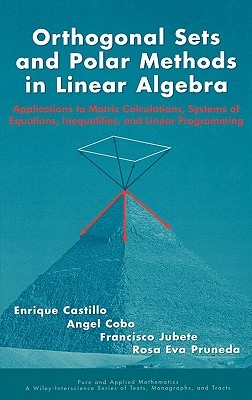Home » Orthogonal Sets and Polar Methods in Linear Algebra: Applications to Matrix Calculations, Systems of Equations, Inequalities, and Linear Programming by Enrique Castillo# Orthogonal Sets and Polar Methods in Linear Algebra: Applications to Matrix Calculations, Systems of Equations, Inequalities, and Linear Programming

## Enrique Castillo

Published February 8th 1999
ISBN : 9780471328896
Hardcover
422 pages
Book Rating:Enter the sum

 About the Book A first-year graduate text on linear algebra for applications, this book is divided into four parts. The first part deals with linear spaces, matrices, systems of equations (including the problems of compatibility and uniqueness), and obtaining theirMoreA first-year graduate text on linear algebra for applications, this book is divided into four parts. The first part deals with linear spaces, matrices, systems of equations (including the problems of compatibility and uniqueness), and obtaining their solutions. The second part deals with cones and systems of linear equalities and inequalities in unrestricted, restricted and strictly restricted variables. The third part presents linear programming problems and the new exterior-point method. The fourth presents a wide collection of examples from the areas of mathematics, physics, and engineering, such as the matrix analysis of structures, input-output tables, and diet and network flow problems. All models presented are taken from real-life problems.